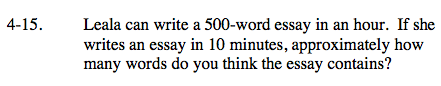### Home > CAAC > Chapter 4 > Lesson 4.1.2 > Problem4-15

4-15.Change hours to minutes.

1 hour = 60 minutes

Use proportions to solve the problem. Assign the variable t to represent the unknown number of words.

$\frac{500\text{ words}}{60\text{ minutes}} = \frac{t\text{ words}}{10\text{ minutes}}$

You can simplify the left side of the proportion and make the denominator 10 by dividing the numerator and denominator by 6. This will also be the answer.